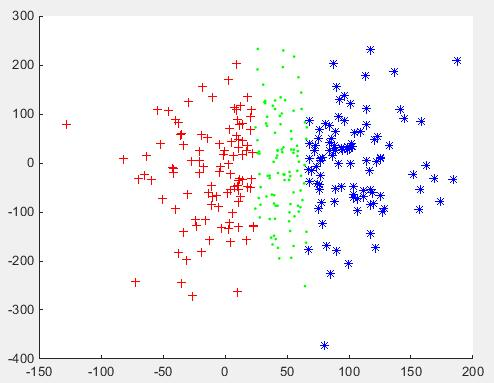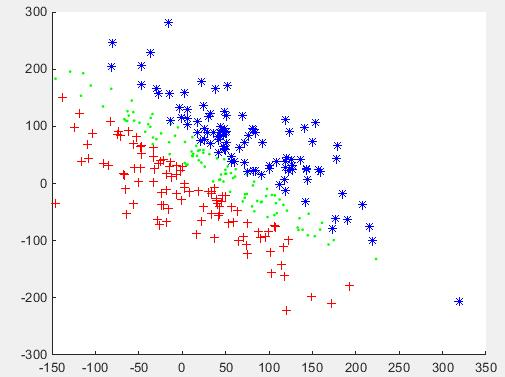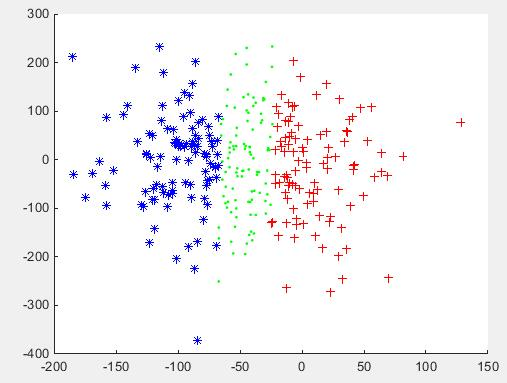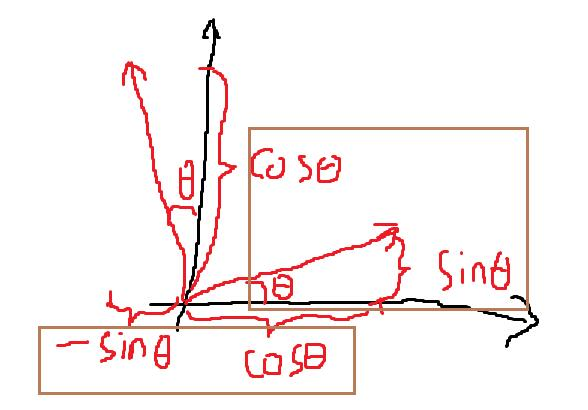主成份分析图解rot=[cos(θ),sin(θ);sin(θ),cos(θ)]启发：

2.在二维平面中，旋转矩阵其实就是新定义的两个特征向量的方向，不用死记，画个图就记住了。

rot=[cos(θ),sin(θ);        sin(θ),cos(θ)]

cos(θ),sin(θ),sin(θ),cos(θ)